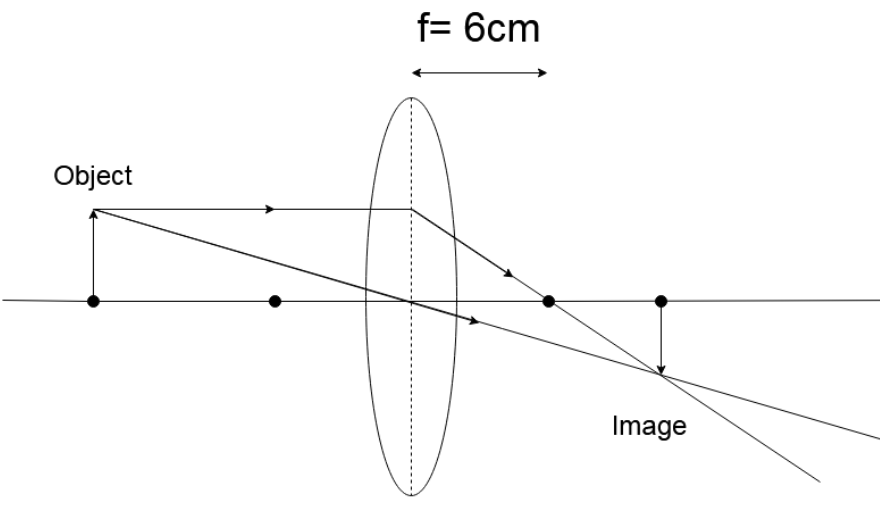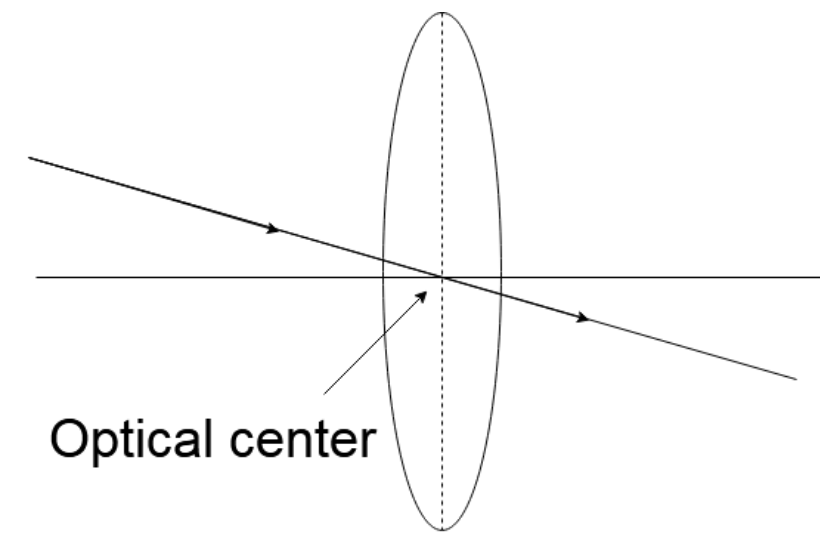(i). A lens produces a magnification of $- 0.5$ . Is this a converging or diverging lens? If the focal length of the lens is $6cm$, draw a ray diagram showing the image formation in this case.(ii). A girl was playing with a thin beam of light from a laser torch by directing it from different directions on a convex lens vertically. She was surprised to see that in a particular direction, the beam of light continued to move along the same direction after passing through the lens. State the reason for her observation. Draw a ray diagram to support your answer.Verified
82.3k+ views
Hint: You can start by writing down the nature of the lens by looking at the sign of the magnification (negative means a convex/converging lens). Then use the equation $m = \dfrac{v}{{\left( { - u} \right)}}$ to get a relation between $v$ and $u$ . Then put these values in the equation $\dfrac{1}{f} = \dfrac{1}{v} - \dfrac{1}{u}$ . Use the value of $v$ and $u$ obtained to make a diagram. Then in the second part, you have to mention where the light must strike the lens in to not get refracted (at the optical center) and then draw a corresponding diagram.

Complete step-by-step solution:
(i). We are given that the lens produces a magnification of $- 0.5$. We know that a concave lens does not have a negative magnification and only convex lenses have negative magnification. A convex lens is converging in nature.
Hence, the given lens is converging.
We are given in the problem
$f = + 6cm$
$m = - 0.5$
We know that,
$m = \dfrac{v}{{\left( { - u} \right)}} = - 0.5$
$v = + 0.5u$
We know by the lens formula
$\dfrac{1}{f} = \dfrac{1}{v} - \dfrac{1}{u}$
$\Rightarrow \dfrac{1}{6} = \dfrac{1}{{0.5u}} - \dfrac{1}{{\left( { - u} \right)}}$
$\Rightarrow \dfrac{1}{6} = \dfrac{1}{{0.5u}} + \dfrac{1}{{\left( { u} \right)}}$
Now on taking LCM,
$\Rightarrow \dfrac{1}{6} = \dfrac{1+0.5}{{0.5u}}$
$\Rightarrow \dfrac{1}{6} = \dfrac{3}{{u}}$
$\Rightarrow u = 18cm$
$\therefore v = 0.5u = 9cm$
The diagram of the given situation is given below(ii). In the problem, we are told that the girl points a laser towards a convex lens that is held vertically. If she points in a particular direction the beam of light does not get refracted. This situation can only occur if the laser is directly pointed at the optical center. Any light ray that strikes at the optical center passes through without any refraction.
The diagram of the given situation is given belowNote: In the first part of the solution above, we mentioned that you can tell whether the lens is convex or concave (hence converging or diverging) by looking at the sign of the magnification. Negative magnification means a convex lens. You could have also predicted nature by looking at the sign of the focal length as the only convex lens has a positive focal length, but we did not do so because here the sign of the focal length was not provided.